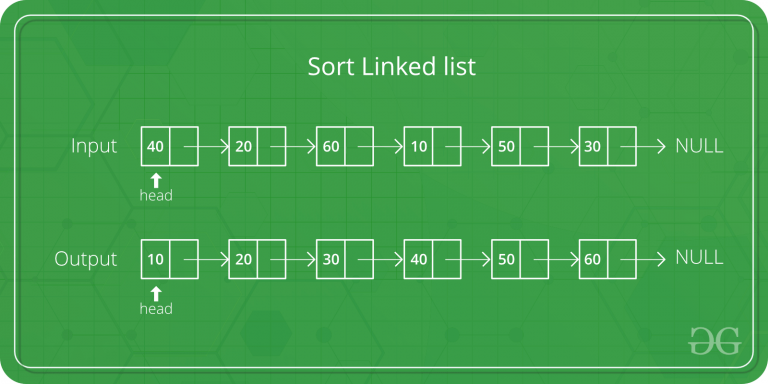# C Program for Bubble Sort on Linked List

Given a singly linked list, sort it using bubble sort.```Input : 10->30->20->5
Output : 5->10->20->30

Input : 20->4->3
Output : 3->4->20```

 `// C program to implement Bubble Sort on singly linked list ` `#include ` `#include ` ` `  `/* structure for a node */` `struct` `Node ` `{ ` `    ``int` `data; ` `    ``struct` `Node *next; ` `}; ` ` `  `/* Function to insert a node at the beginning of a linked list */` `void` `insertAtTheBegin(``struct` `Node **start_ref, ``int` `data); ` ` `  `/* Function to bubble sort the given linked list */` `void` `bubbleSort(``struct` `Node *start); ` ` `  `/* Function to swap data of two nodes a and b*/` `void` `swap(``struct` `Node *a, ``struct` `Node *b); ` ` `  `/* Function to print nodes in a given linked list */` `void` `printList(``struct` `Node *start); ` ` `  `int` `main() ` `{ ` `    ``int` `arr[] = {12, 56, 2, 11, 1, 90}; ` `    ``int` `list_size, i; ` ` `  `    ``/* start with empty linked list */` `    ``struct` `Node *start = NULL; ` ` `  `    ``/* Create linked list from the array arr[]. ` `      ``Created linked list will be 1->11->2->56->12 */` `    ``for` `(i = 0; i< 6; i++) ` `        ``insertAtTheBegin(&start, arr[i]); ` ` `  `    ``/* print list before sorting */` `    ``printf``(``"\n Linked list before sorting "``); ` `    ``printList(start); ` ` `  `    ``/* sort the linked list */` `    ``bubbleSort(start); ` ` `  `    ``/* print list after sorting */` `    ``printf``(``"\n Linked list after sorting "``); ` `    ``printList(start); ` ` `  `    ``getchar``(); ` `    ``return` `0; ` `} ` ` `  ` `  `/* Function to insert a node at the beginning of a linked list */` `void` `insertAtTheBegin(``struct` `Node **start_ref, ``int` `data) ` `{ ` `    ``struct` `Node *ptr1 = (``struct` `Node*)``malloc``(``sizeof``(``struct` `Node)); ` `    ``ptr1->data = data; ` `    ``ptr1->next = *start_ref; ` `    ``*start_ref = ptr1; ` `} ` ` `  `/* Function to print nodes in a given linked list */` `void` `printList(``struct` `Node *start) ` `{ ` `    ``struct` `Node *temp = start; ` `    ``printf``(``"\n"``); ` `    ``while` `(temp!=NULL) ` `    ``{ ` `        ``printf``(``"%d "``, temp->data); ` `        ``temp = temp->next; ` `    ``} ` `} ` ` `  `/* Bubble sort the given linked list */` `void` `bubbleSort(``struct` `Node *start) ` `{ ` `    ``int` `swapped, i; ` `    ``struct` `Node *ptr1; ` `    ``struct` `Node *lptr = NULL; ` ` `  `    ``/* Checking for empty list */` `    ``if` `(start == NULL) ` `        ``return``; ` ` `  `    ``do` `    ``{ ` `        ``swapped = 0; ` `        ``ptr1 = start; ` ` `  `        ``while` `(ptr1->next != lptr) ` `        ``{ ` `            ``if` `(ptr1->data > ptr1->next->data) ` `            ``{  ` `                ``swap(ptr1, ptr1->next); ` `                ``swapped = 1; ` `            ``} ` `            ``ptr1 = ptr1->next; ` `        ``} ` `        ``lptr = ptr1; ` `    ``} ` `    ``while` `(swapped); ` `} ` ` `  `/* function to swap data of two nodes a and b*/` `void` `swap(``struct` `Node *a, ``struct` `Node *b) ` `{ ` `    ``int` `temp = a->data; ` `    ``a->data = b->data; ` `    ``b->data = temp; ` `} `

Output:

``` Linked list before sorting
90 1 11 2 56 12